# PMT Varying Rates

This page demonstrates the repayment function with varying interest rates. The PMT or the PPMT functions do not work when the interest varies.  With the varying repayment function, you can enter a series of interest rates and a debt tenure and the function produces the repayment percent. To simply enter a function on a the debt tenure and a series of cash flows and find the repayment percent. You use the function =repayment_varying_rate(tenure, interest rate series, optional debt repayment flag).  This function is an array function meaning that you have to paint the area where the repayment percent will go.  Technical details below demonstrate how to create the UDF function with VBA code by computing the remaining tenure and the remaining cash flow and then evaluating the PMT for a loan of 1.0.

## Using the Repayment with Varying Interest Rates UDF

Once you have imported the repayment with varying interest rates UDF function, you can use the =repayment_varying_rate(tenure, interest rate series, optional debt repayment flag) function  as shown in the screenshot below. In the example, I have made a simple debt balance and a debt tenure of 20 years.  The example shows that you can click on the entire line to get the repayment percent (the final screenshot). The screenshot below is an illustration of the most basic case for the repayment function.  There is no debt repayment flag and the interest rate is select rather than the whole line.  Don’t forget to press SHIFT, CNTL, ENTER after you have copied the function to the entire row.  (You can copy the function first, then press F2, and then press SHIFT, CNTL, ENTER).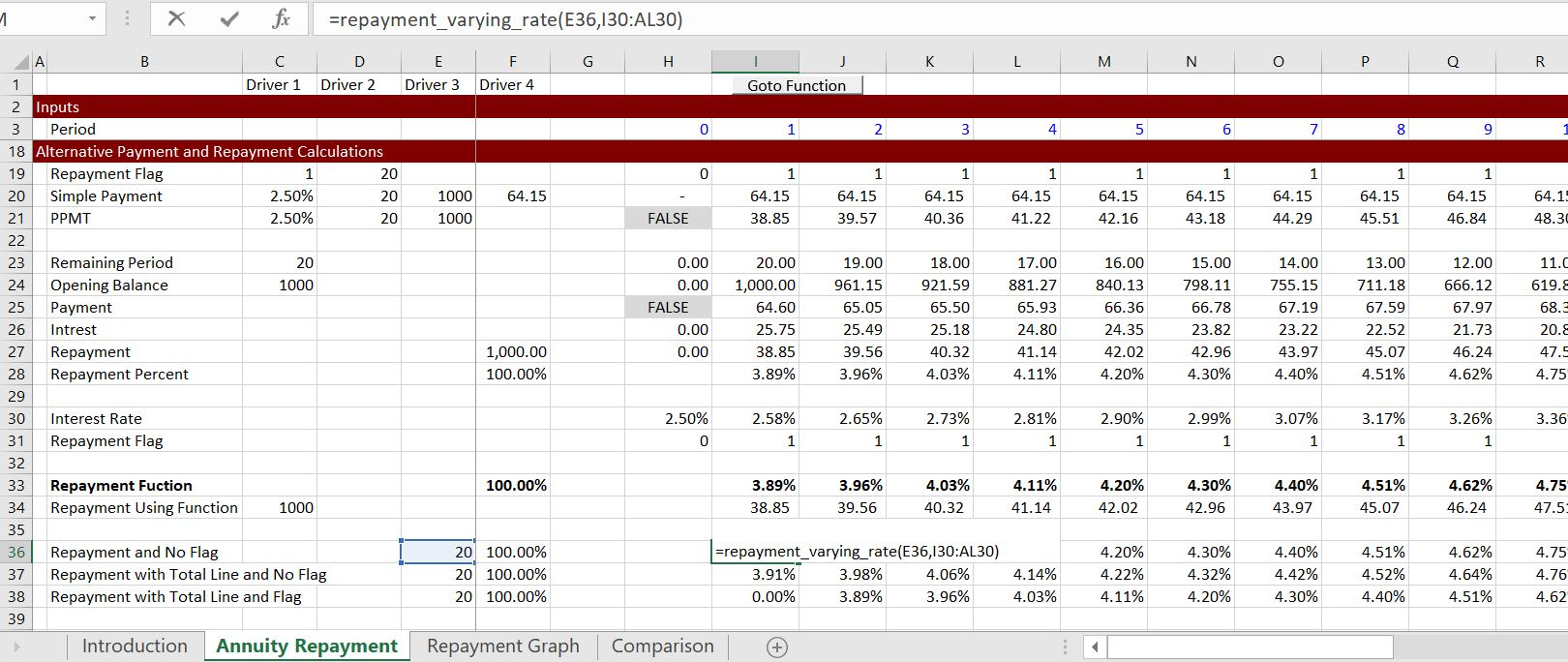The screenshot below illustrates use of the function when the option flag is not used.  This illustrates that you do not have to use the optional flag and you can use the entire line.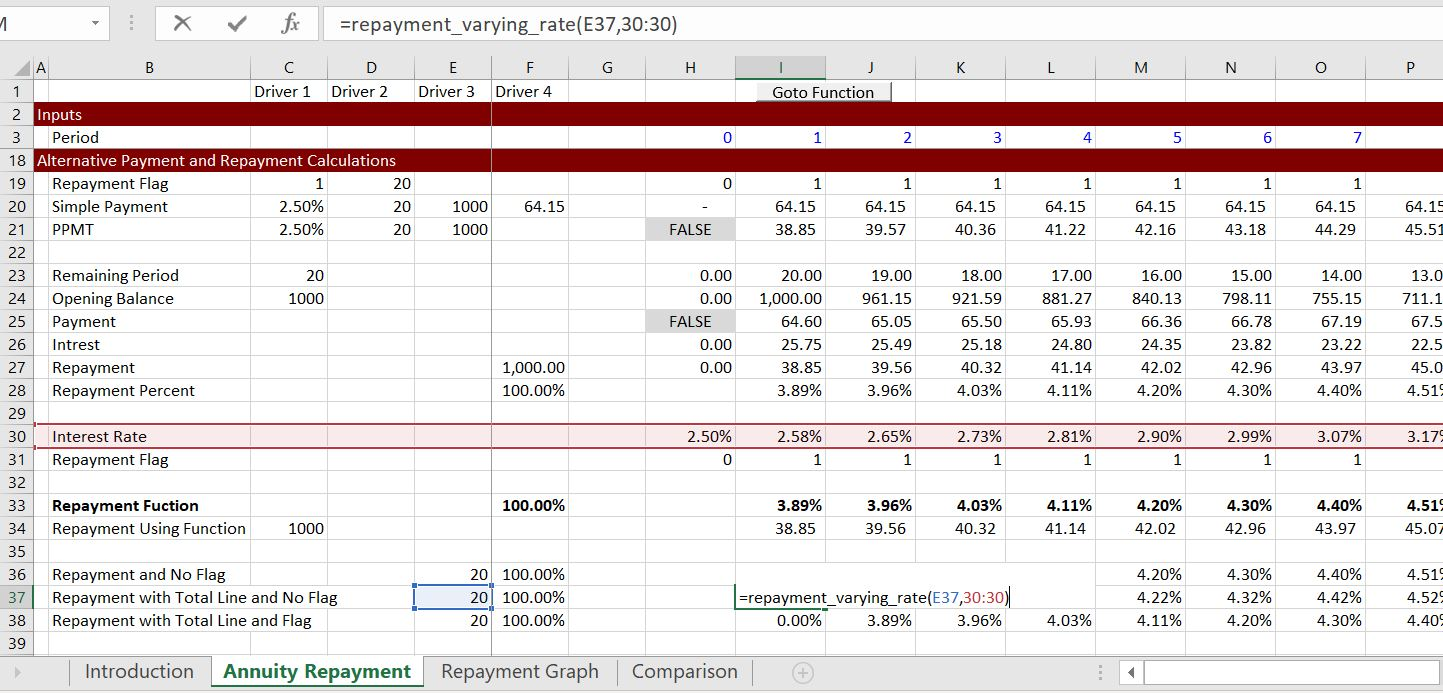The final screenshot shows the case where the entire line for the interest rate and the entire line for the flag is read in.  This is by far the most useful case for real models.

## Inserting the Varying Repayment Function into a Your Workbook

After you open the file that is available for download above you can do a few things to get the function into your sheet.  One is to press the button that will produce a user form.  Then, in the text that comes up, just select the VBA code and copy it to your sheet (as shown in the screenshot below the file).  Alternatively, you can press the ALT and F8 key sequence, edit the VBA code, press CNTL, C and then copy it to your file.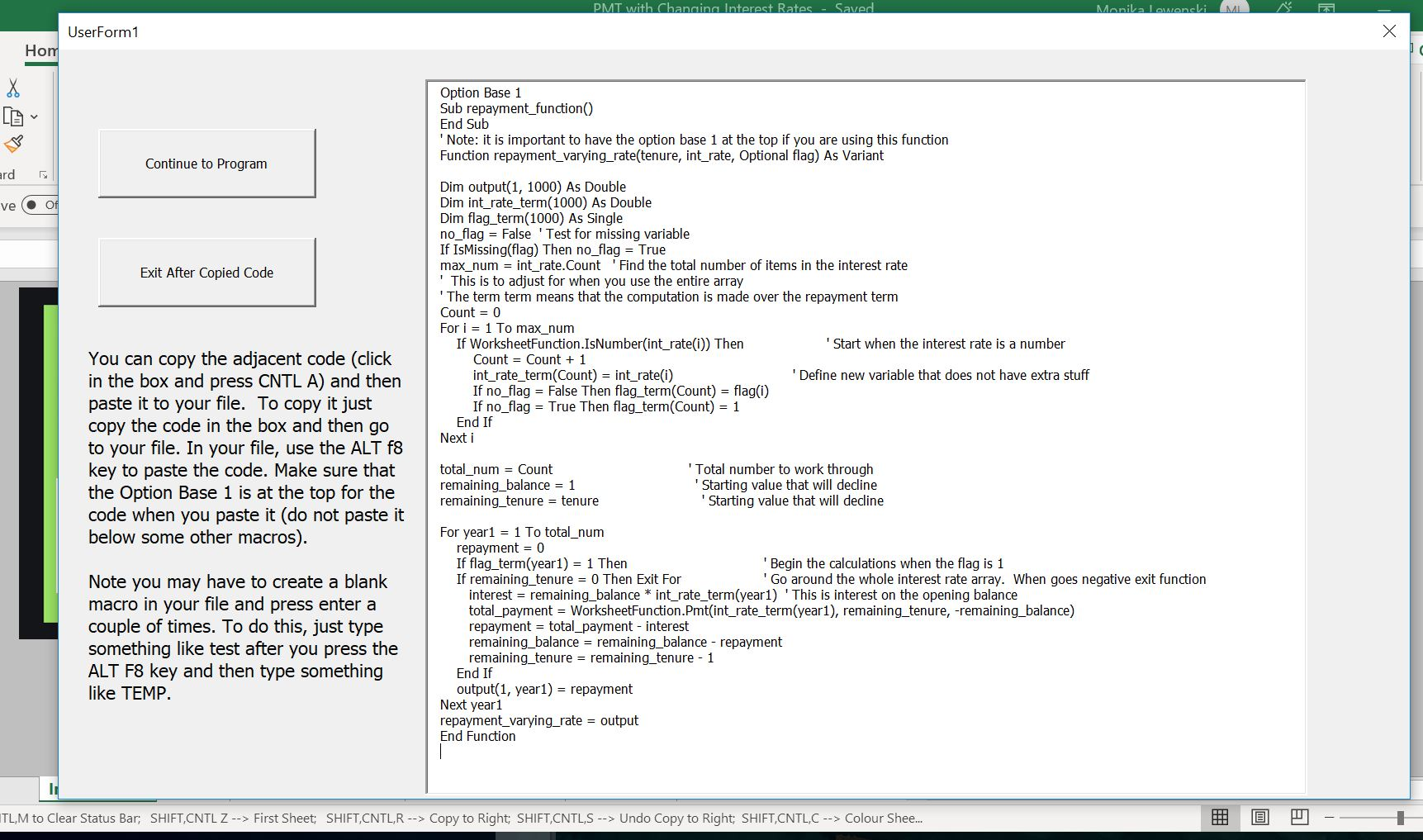Once you have copied the VBA code (either from the userform, from pressing ALT, F8 and copying the entire sheet or from copying the code below), you can copy it into your file.  To do this, you can press ALT, F8 to get the VBA screen.  Then put in any name (e.g. Stormy).  Then go to the top of the file and copy the code (CNTL, V).  Some of this is demonstrated in the couple of  screenshots below.  The first screen shot illustrates how to create a new VBA page after you press ALT, F8; the second shows how you should insert a line to put the code at the top of the page; the third shows the results after you copy.After making the new page with you blank macro named Stormy, go above the new macro and enter a couple of blank lines.  When you have entered the blank lines you will press the CNTL, V and copy the code to the top of the page.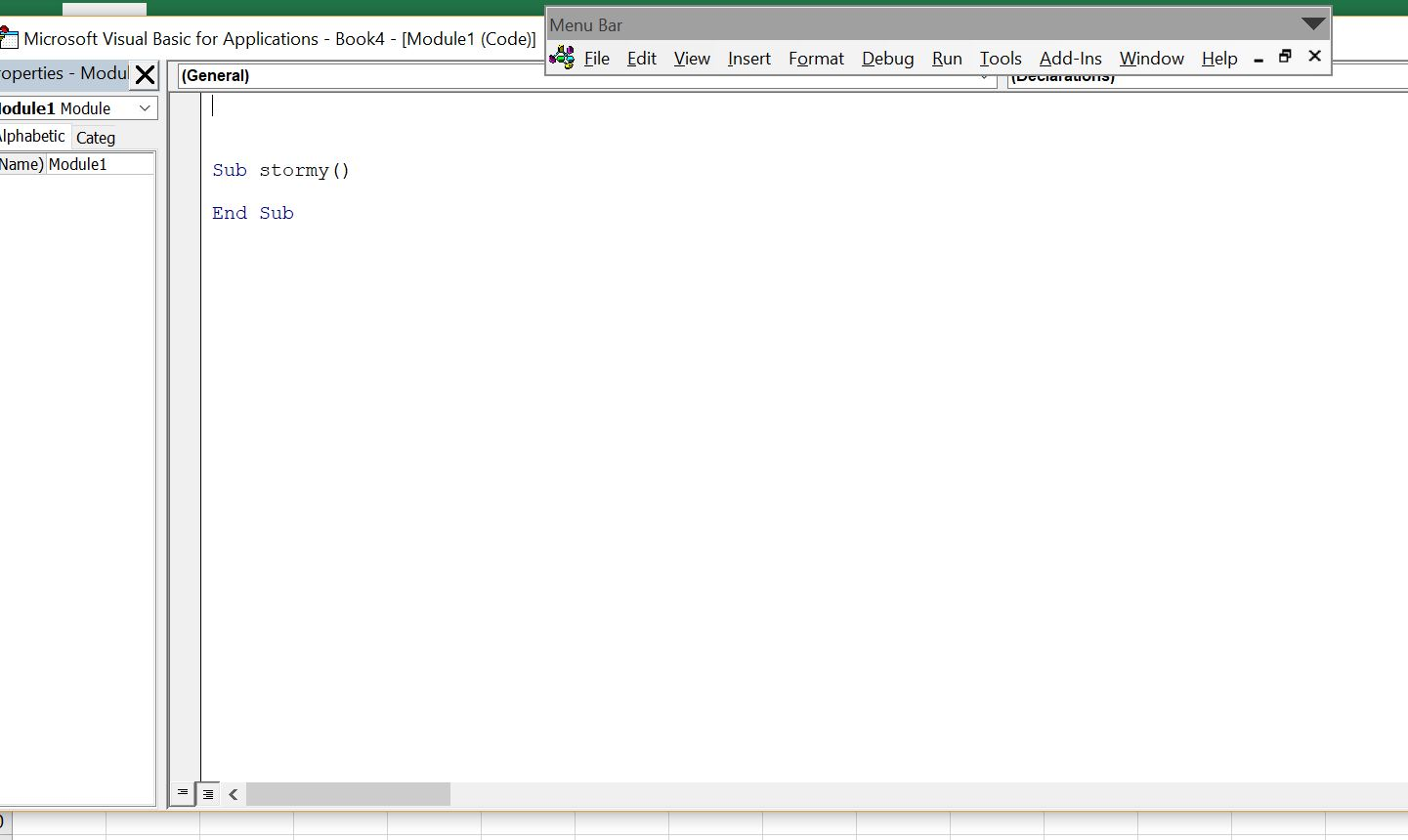After you have copied the code into your sheet, you should see OPTION BASE 1 at the top of the page.  Now you are ready to go and the function should work in your workbook.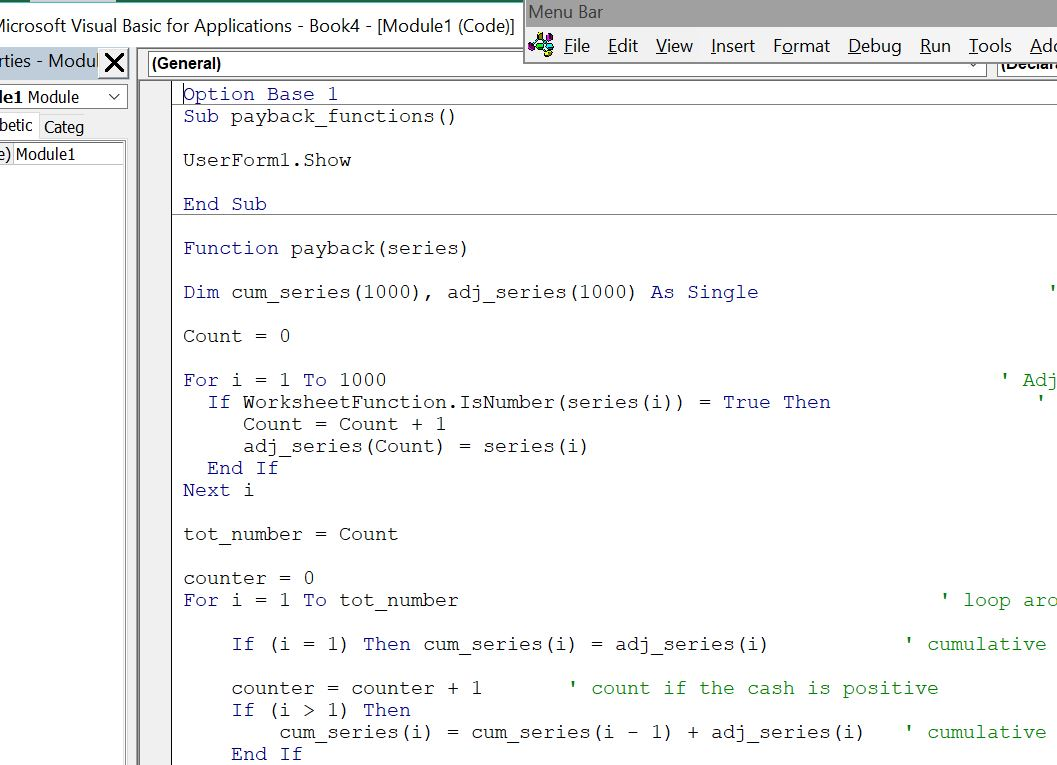## Creating the Function for Annuity Repayment with Changing Interest Rates

The user defined function for creating a varying repayment is presented below.  You can see the adjustment required so that an entire row can be used and you can see how the loop works with counting the number of cash flows.

.

```Option Base 1
Sub repayment_function()
End Sub

' Note: it is important to have the option base 1 at the top if you are using this function

Function repayment_varying_rate(tenure, int_rate, Optional flag) As Variant

Dim output(1, 1000) As Double
Dim int_rate_term(1000) As Double
Dim flag_term(1000) As Single

no_flag = False ' Test for missing variable

If IsMissing(flag) Then no_flag = True

max_num = int_rate.Count ' Find the total number of items in the interest rate

' This is to adjust for when you use the entire array
' The term term means that the computation is made over the repayment term

Count = 0
For i = 1 To max_num

If WorksheetFunction.IsNumber(int_rate(i)) Then ' Start when the interest rate is a number
Count = Count + 1
int_rate_term(Count) = int_rate(i) ' Define new variable that does not have extra stuff
If no_flag = False Then flag_term(Count) = flag(i)
If no_flag = True Then flag_term(Count) = 1
End If
Next i

total_num = Count ' Total number to work through
remaining_balance = 1 ' Starting value that will decline
remaining_tenure = tenure ' Starting value that will decline

For year1 = 1 To total_num
repayment = 0

If flag_term(year1) = 1 Then ' Begin the calculations when the flag is 1
If remaining_tenure = 0 Then Exit For ' Go around the whole interest rate array. When goes negative exit function
interest = remaining_balance * int_rate_term(year1) ' This is interest on the opening balance
total_payment = WorksheetFunction.Pmt(int_rate_term(year1), remaining_tenure, -remaining_balance)
repayment = total_payment - interest
remaining_balance = remaining_balance - repayment
remaining_tenure = remaining_tenure - 1
End If
output(1, year1) = repayment
Next year1

repayment_varying_rate = output

End Function

.```

The function below is very similar, but it includes discounting the cash flow. This discounting can be

.

## Video Describing How to Create Repayment Function with Varying Interest Rates

If all of the above explanation does not do enough, then you can watch a video on how to create a payback function.